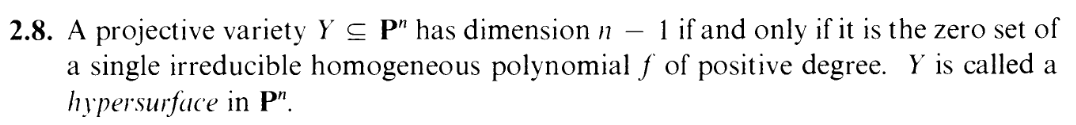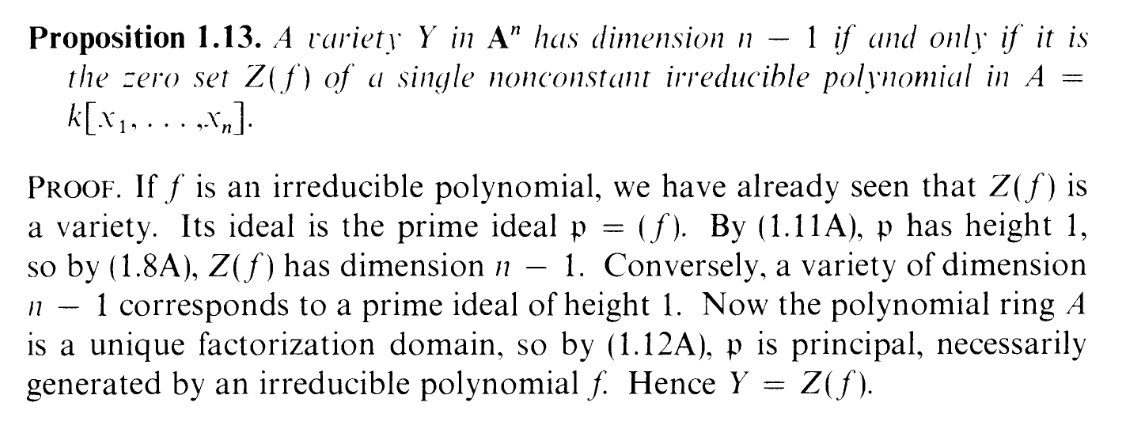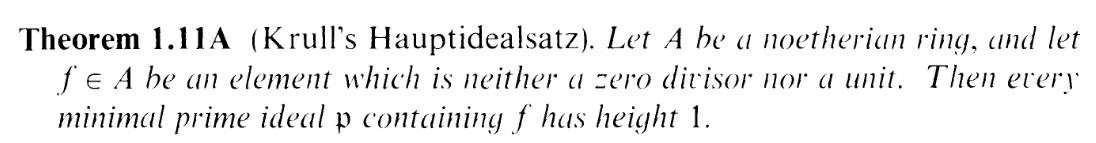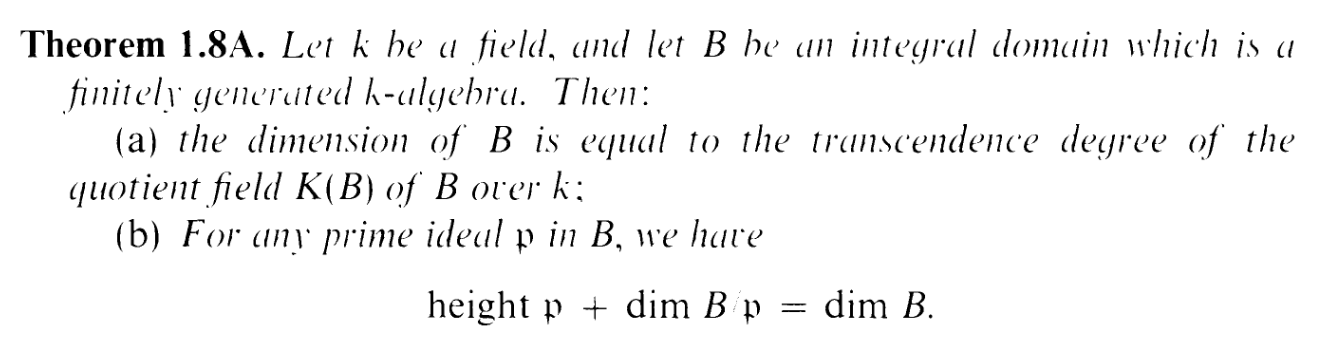I.2.8

2/9/2021Welll... Might as well quickly crank out another one before I go to bed. It's yet another projectivization of affine question. Here's the affine version:And unlike some of the trickier exercises like the last two, we can just follow the affine proof for this one. Here's Theorem 1.11A, which we'll use:And also Theorem 1.8A:Since Y is a variety, note that I(Y ) is prime. And here's my slick proof:

 dim Y = n - 1 ⇐⇒ dim Y + 1 = n (Adding 1 to both sides) ⇐⇒ dim S(Y ) = n (by 2.6) ⇐⇒ dim k[x0,…,xn] - htI(Y ) = n (by 1.8A) ⇐⇒n + 1 - htI(Y ) = n ⇐⇒htI(Y ) = 1 ⇐⇒I(Y ) = (f) where f satisfies the requirements
That last equality is thanks to 1.11A and observing that in UFDs, f is prime iff it is irreducible. (Yes I put this sentence outside of the align environment to prevent it from going outside my post because I still haven't figure out how to fix that FffFFFFFFFFFFFFFFFffFUUUUUGG)